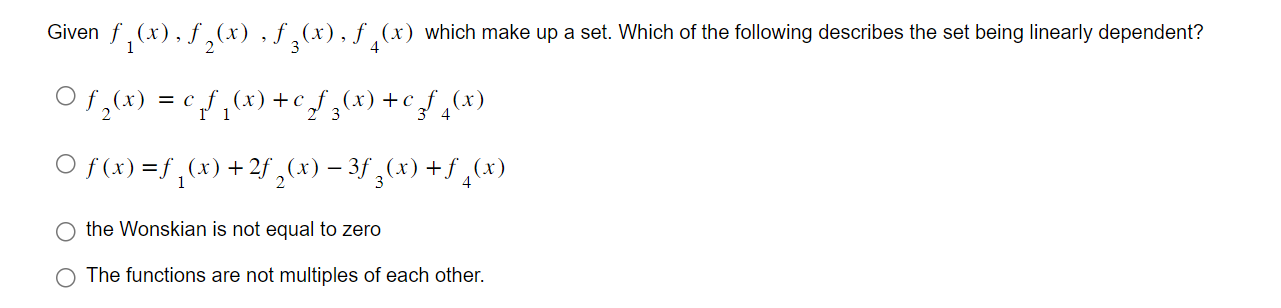# Question Given $$f_{1}(x), f_{2}(x), f_{3}(x), f_{4}(x)$$ which make up a set. Which of the following describes the set being linearly dependent? $\begin{array}{l} f_{2}(x)=c_{1} f_{1}(x)+c_{2} f_{3}(x)+c_{3} f_{4}(x) \\ f(x)=f_{1}(x)+2 f_{2}(x)-3 f_{3}(x)+f_{4}(x) \end{array}$ the Wonskian is not equal to zero The functions are not multiples of each other.Transcribed Image Text: Given $$f_{1}(x), f_{2}(x), f_{3}(x), f_{4}(x)$$ which make up a set. Which of the following describes the set being linearly dependent? $\begin{array}{l} f_{2}(x)=c_{1} f_{1}(x)+c_{2} f_{3}(x)+c_{3} f_{4}(x) \\ f(x)=f_{1}(x)+2 f_{2}(x)-3 f_{3}(x)+f_{4}(x) \end{array}$ the Wonskian is not equal to zero The functions are not multiples of each other.
Transcribed Image Text: Given $$f_{1}(x), f_{2}(x), f_{3}(x), f_{4}(x)$$ which make up a set. Which of the following describes the set being linearly dependent? $\begin{array}{l} f_{2}(x)=c_{1} f_{1}(x)+c_{2} f_{3}(x)+c_{3} f_{4}(x) \\ f(x)=f_{1}(x)+2 f_{2}(x)-3 f_{3}(x)+f_{4}(x) \end{array}$ the Wonskian is not equal to zero The functions are not multiples of each other.&#12304;General guidance&#12305;The answer provided below has been developed in a clear step by step manner.Step1/2Since given function $$\mathrm{{{f}_{{1}}{\left({x}\right)}},{{f}_{{2}}{\left({x}\right)}},{{f}_{{{3}}}{\left({x}\right)}},{{f}_{{4}}{\left({x}\right)}}}$$ are linearly dependent then option 1 will be true.ExplanationA set of vector is linearly dependent if one of them can be written as linear ... See the full answer